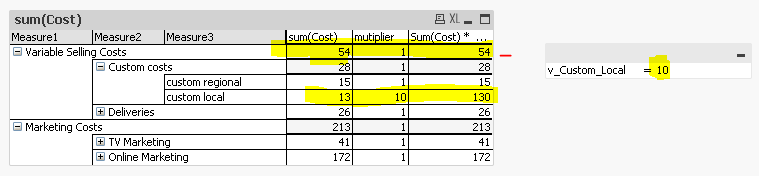# QlikView App Development

Discussion Board for collaboration related to QlikView App Development.

New Contributor III

## Simulating values in a pivot table

I have  a set of costs represented in pivot table.  I want to simulate  "custom local" -- which is ok. BUt how can I get that simulated value to effect the total sum of "variable selling costs"?   the qlikview pivot table dismisses any modificaions made using IF function and calculates total sum value the initial input

I am successfully able to estimate/simulate "custom local" by multiplying it with a variable using a if function.

Sum(Cost) *  if(Measure3 ='custom local',v_Custom_Local, 1)

but it doesnt get included in the total sum?     variable selling costs should be 171 and Custom costs should be 145.script is  the following

COST_TABLE:
Measure1,Measure2,Measure3,Cost
Variable Selling Costs,Custom costs,custom regional,15
Variable Selling Costs,Custom costs,custom local,13

];

let v_Custom_Local = 2; // returns the date serial number of today.

Tags (6)
1 Solution

Accepted Solutions
HighlightedMVP

## Re: Simulating values in a pivot table

May be this?

Sum(Aggr(Sum(Cost) *  if(Measure3 ='custom local',v_Custom_Local, 1), Measure1. Measure2, Measure3))

Before develop something, think If placed (The Right information | To the right people | At the Right time | In the Right place | With the Right context)